Courses

# Test: Circuit Theory

## 25 Questions MCQ Test Electrical Engineering SSC JE (Technical) | Test: Circuit Theory

Description
This mock test of Test: Circuit Theory for Electrical Engineering (EE) helps you for every Electrical Engineering (EE) entrance exam. This contains 25 Multiple Choice Questions for Electrical Engineering (EE) Test: Circuit Theory (mcq) to study with solutions a complete question bank. The solved questions answers in this Test: Circuit Theory quiz give you a good mix of easy questions and tough questions. Electrical Engineering (EE) students definitely take this Test: Circuit Theory exercise for a better result in the exam. You can find other Test: Circuit Theory extra questions, long questions & short questions for Electrical Engineering (EE) on EduRev as well by searching above.
QUESTION: 1

Solution:
QUESTION: 2

Solution:
QUESTION: 3

### An inductor, at t = 0+. with zero initial current acts as:

Solution:
QUESTION: 4

The condition for the electrical symmetry of the circuit is that

Solution:
QUESTION: 5

At half power frequencies. the current in the RLC series circuit is:

Solution:
QUESTION: 6

The 3dB bandwidth of series RLC tuned circuit is 10 KHz and its frequency of resonance is 19000 KHZ. The Q of the circuit is:

Solution:
QUESTION: 7

In an RLC series circuit, at resonance the impedance is:

Solution:
QUESTION: 8

The formula for determining the inductance of two coils connected in series aiding is

Solution:
QUESTION: 9

The formula for the resonant frequency is f =

Solution:
QUESTION: 10

If f1 and f2 are the 3–dB frequencies and f0 is the frequency of resonance, the the selectivity Q of RLC circuit is given by

Solution:
QUESTION: 11

A source V has an internal impedance Zin = (R + jx). When it is connected to load of ZL + R –jx, the power transferred is

Solution:
QUESTION: 12

Bandwidth of a series resonant circuit is given by

Solution:
QUESTION: 13

In an RLC parallel circuit, the impedance at resonance is:

Solution:
QUESTION: 14

Equivalent resistance of the given network is: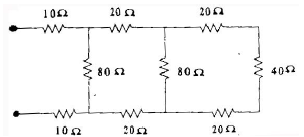Solution:
QUESTION: 15

For a pure capacitor, the voltage current relationship is

Solution:
QUESTION: 16

In the given figure, the Thevenin's equivalent pair (voltage, impedance), as seen at the terminals P – Q, is given by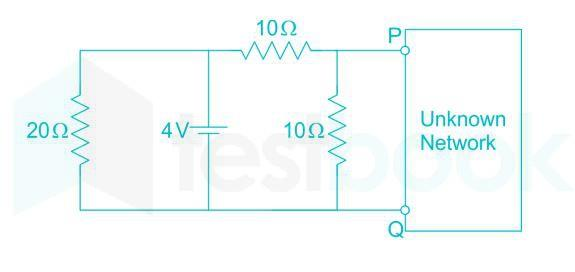Solution: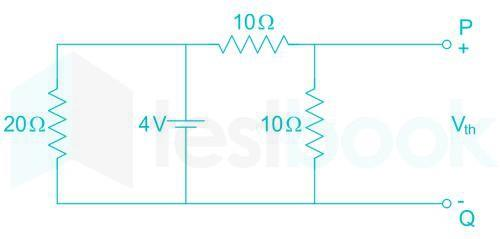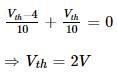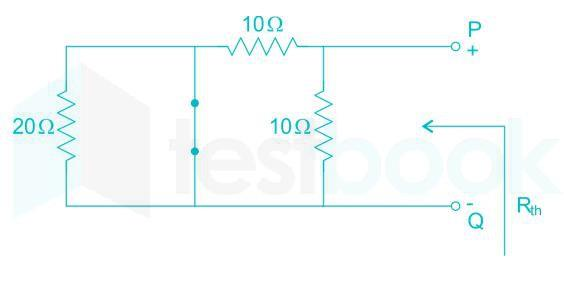QUESTION: 17

Two coupled inductors L1 = 0.2 H and L2 = 0.45 H have coefficient of coupling k = 0.8. The mutual inductance M is.

Solution:
QUESTION: 18

Double energy transients occur in the :

Solution:
QUESTION: 19

The energy stored in a capacitor and inductor is given by

Solution:
QUESTION: 20

For maximum power transfer, according to maximum power transfer theorem, source impedance

Solution:
QUESTION: 21

The equivalent inductance of the given circuit between terminals a–b is equal to :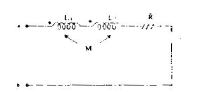Solution:
QUESTION: 22

Time constant of a series R–L circuit is given by:

Solution:
QUESTION: 23

A series resonant circuit has an inductive reactance of 1000W, a capacitive reactance of 1000W and a resistance of 0. 1W. If the resonant frequency is 10 MHz, then the bandwidth of the circuit will be

Solution:
QUESTION: 24

The resistance 'R' looking into the terminals AB in the circuit shown in the figure will be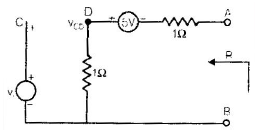Solution:
QUESTION: 25

In the circuit shown in the figure, the power dissipated in 30W resistor will be maximum if the value of R is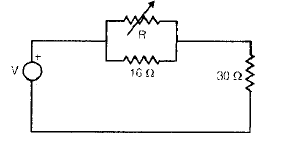Solution: